Examples

Chapter 9 Class 11 Sequences and Series
Serial order wise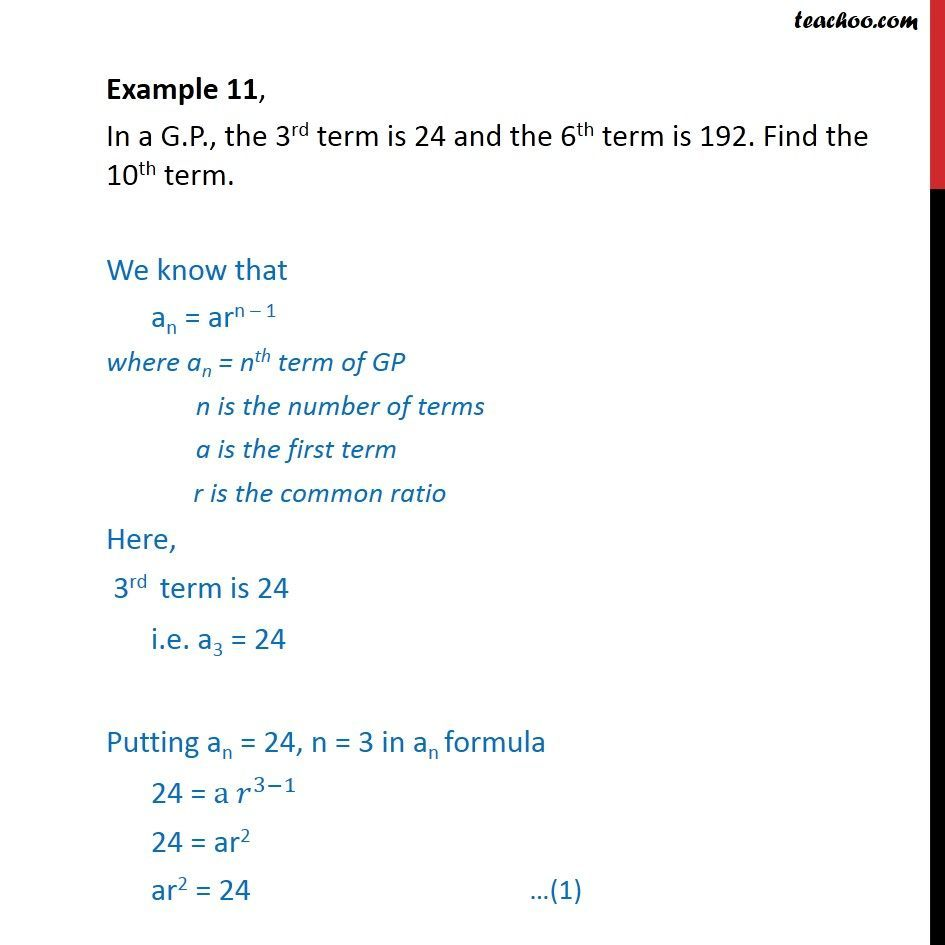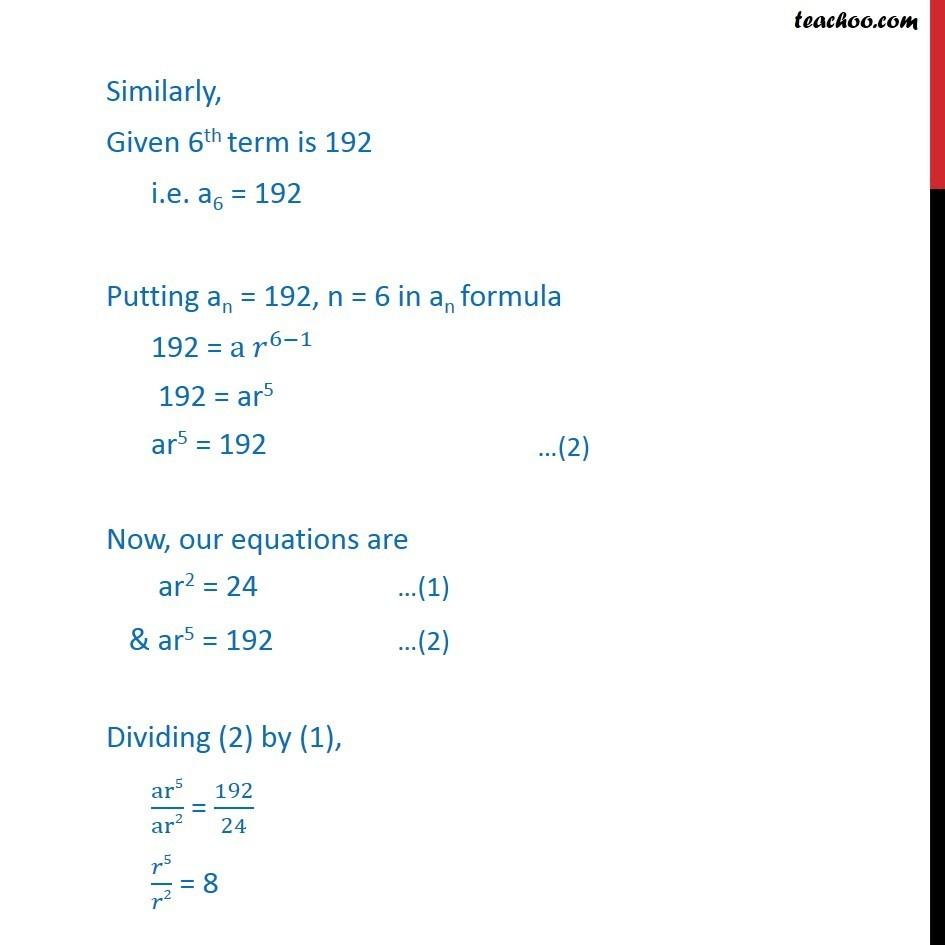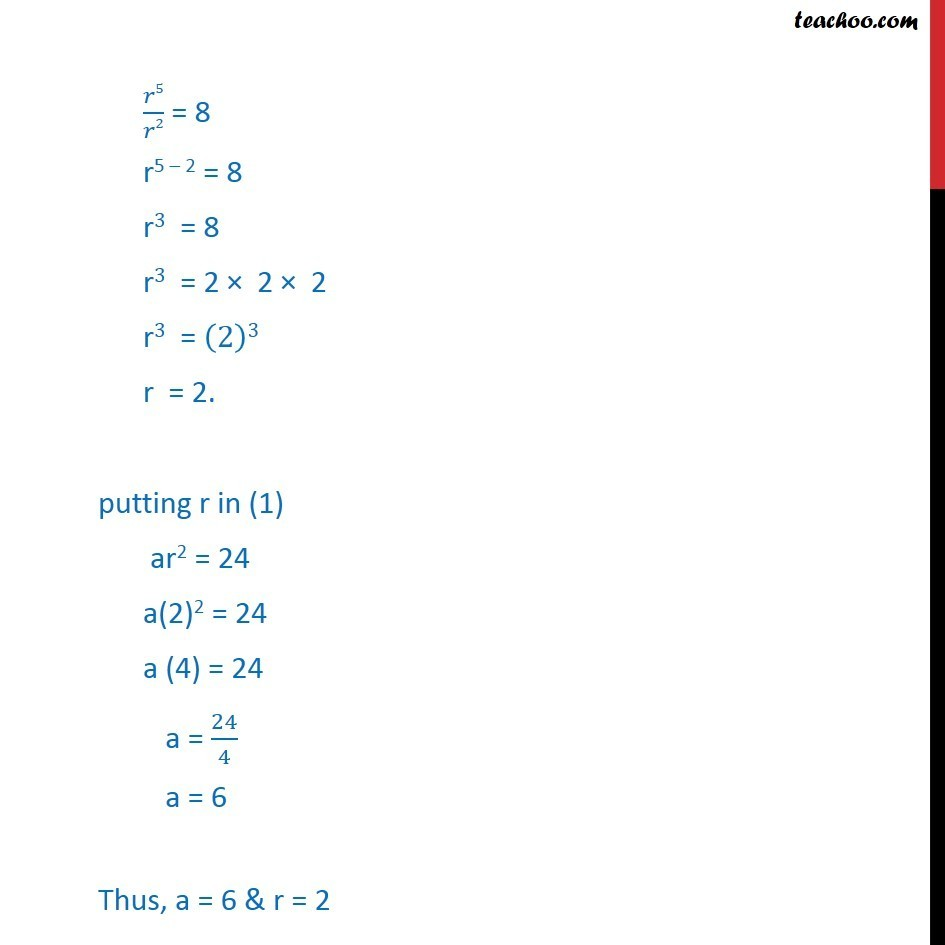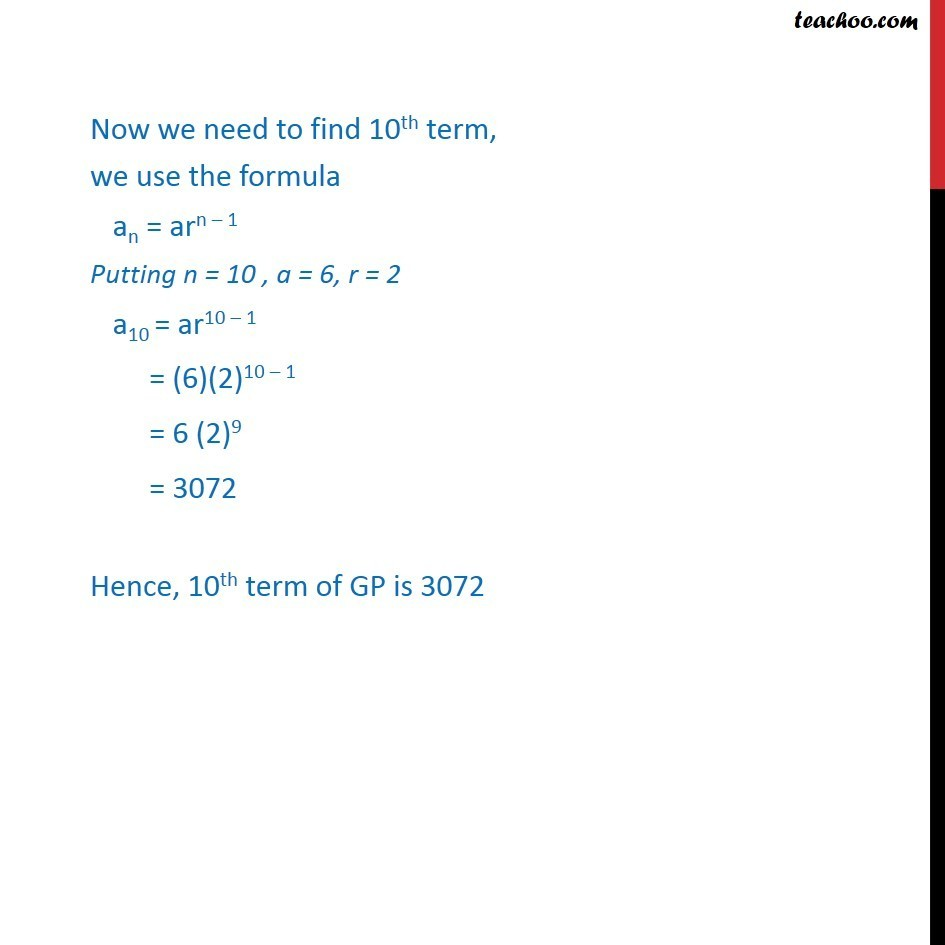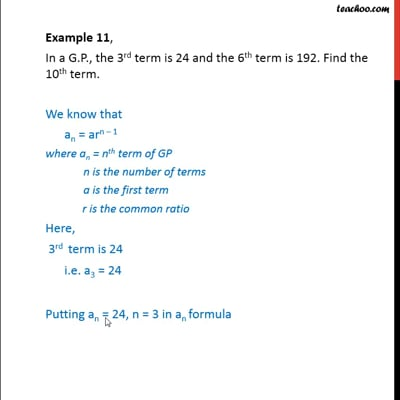This video is only available for Teachoo black users

Solve all your doubts with Teachoo Black (new monthly pack available now!)

### Transcript

Example 11, In a G.P., the 3rd term is 24 and the 6th term is 192. Find the 10th term. We know that an = arn – 1 where an = nth term of GP n is the number of terms a is the first term r is the common ratio Here, 3rd term is 24 i.e. a3 = 24 Putting an = 24, n = 3 in an formula 24 = a 𝑟^(3−1) 24 = ar2 ar2 = 24 Similarly, Given 6th term is 192 i.e. a6 = 192 Putting an = 192, n = 6 in an formula 192 = a 𝑟^(6−1) 192 = ar5 ar5 = 192 Now, our equations are ar2 = 24 …(1) & ar5 = 192 …(2) Dividing (2) by (1), ar5/ar2 = 192/24 𝑟5/𝑟2 = 8 𝑟5/𝑟2 = 8 r5 – 2 = 8 r3 = 8 r3 = 2 × 2 × 2 r3 = (2)3 r = 2. putting r in (1) ar2 = 24 a(2)2 = 24 a (4) = 24 a = 24/4 a = 6 Thus, a = 6 & r = 2 Now we need to find 10th term, we use the formula an = arn – 1 Putting n = 10 , a = 6, r = 2 a10 = ar10 – 1 = (6)(2)10 – 1 = 6 (2)9 = 3072 Hence, 10th term of GP is 3072